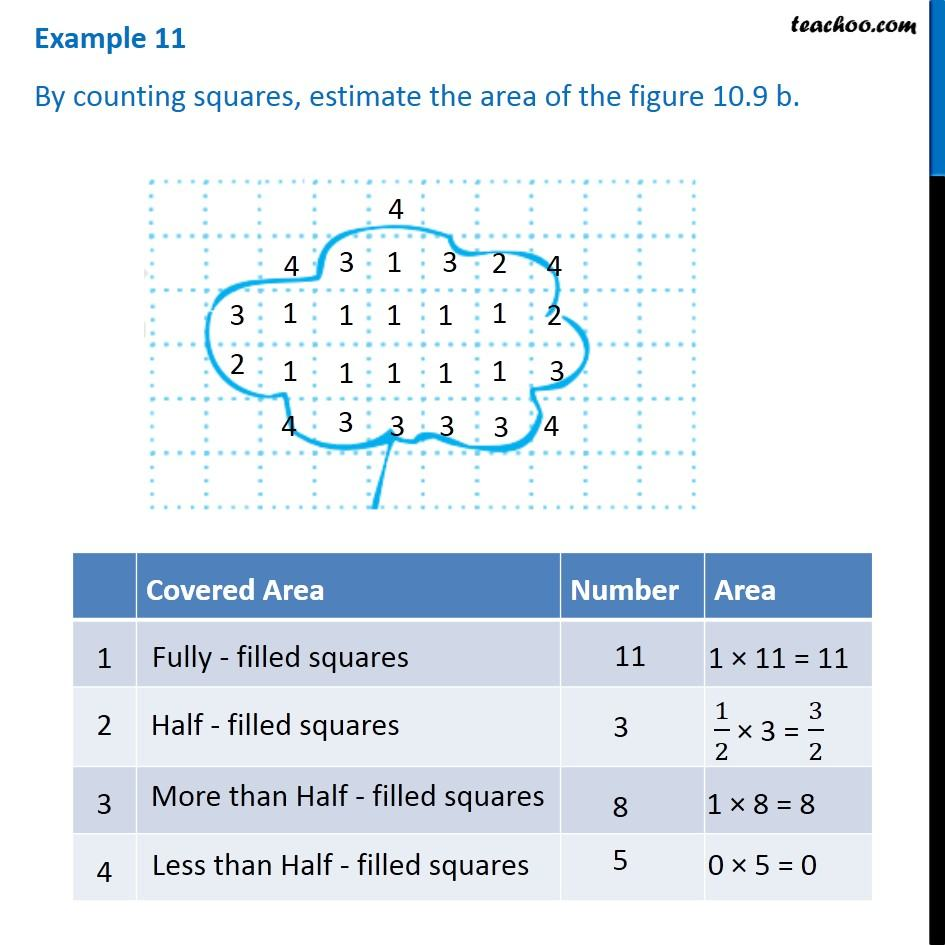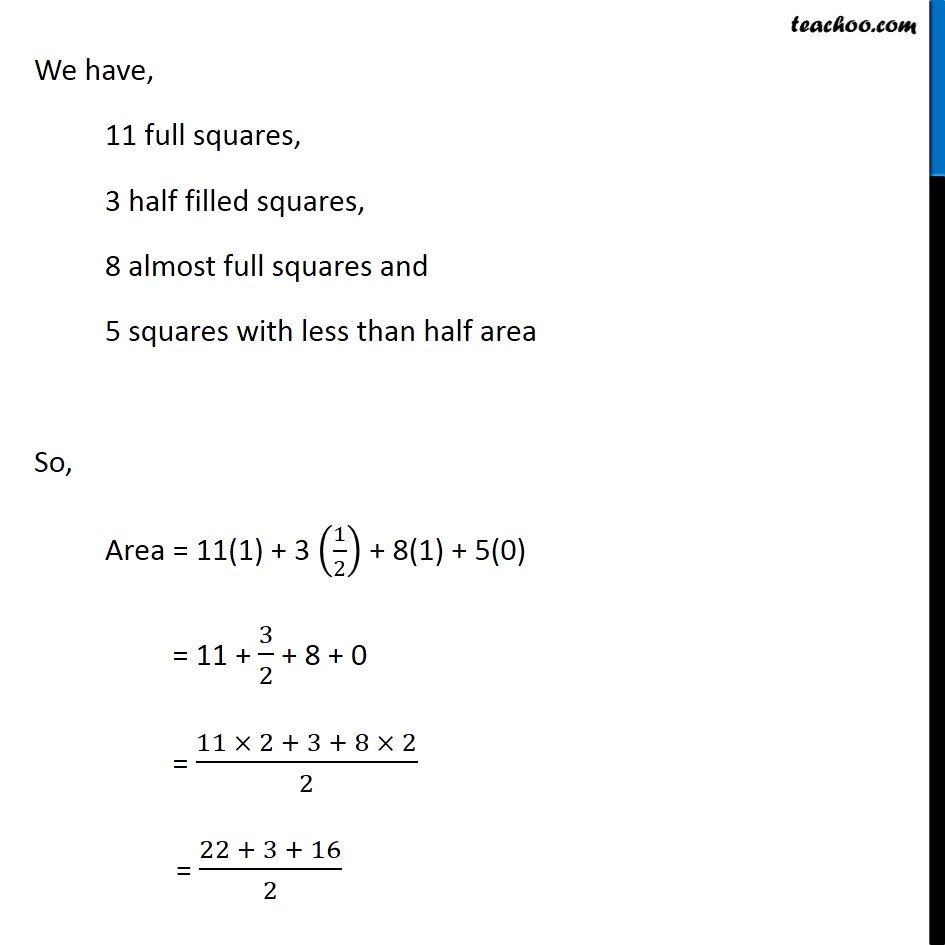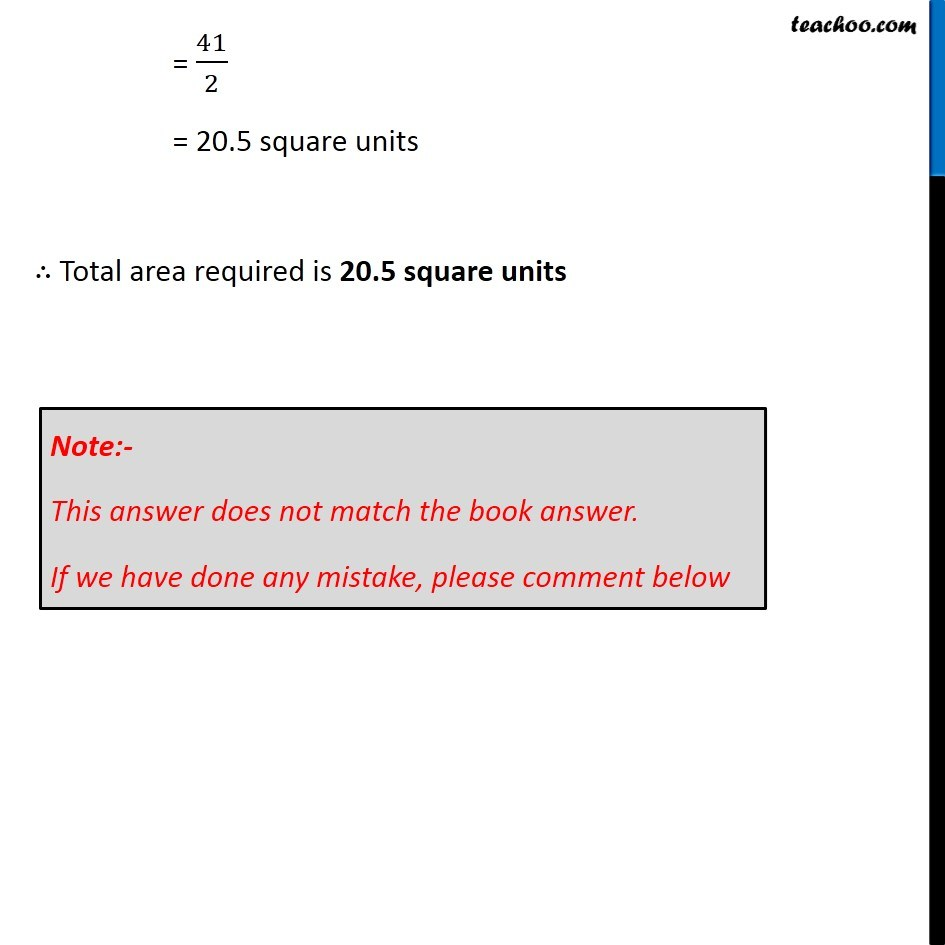Examples

Chapter 10 Class 6 Mensuration
Serial order wiseLearn in your speed, with individual attention - Teachoo Maths 1-on-1 Class

### Transcript

Example 11 By counting squares, estimate the area of the figure 10.9 b. We have, 11 full squares, 3 half filled squares, 8 almost full squares and 5 squares with less than half area So, Area = 11(1) + 3 (1/2) + 8(1) + 5(0) = 11 + 3/2 + 8 + 0 = (11 × 2 + 3 + 8 × 2)/2 = (22 + 3 + 16)/2 = 41/2 = 20.5 square units ∴ Total area required is 20.5 square units Note:- This answer does not match the book answer. If we have done any mistake, please comment below